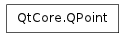QPointF

QElapsedTimer

QPoint¶Detailed Description¶

The PySide.QtCore.QPoint class defines a point in the plane using integer precision.

A point is specified by a x coordinate and an y coordinate which can be accessed using the PySide.QtCore.QPoint.x() and PySide.QtCore.QPoint.y() functions. The PySide.QtCore.QPoint.isNull() function returns true if both x and y are set to 0. The coordinates can be set (or altered) using the PySide.QtCore.QPoint.setX() and PySide.QtCore.QPoint.setY() functions, or alternatively the PySide.QtCore.QPoint.rx() and PySide.QtCore.QPoint.ry() functions which return references to the coordinates (allowing direct manipulation).

Given a point p , the following statements are all equivalent:

p = QPoint()

p.setX(p.x() + 1)
p += QPoint(1, 0)

A PySide.QtCore.QPoint object can also be used as a vector: Addition and subtraction are defined as for vectors (each component is added separately). A PySide.QtCore.QPoint object can also be divided or multiplied by an int or a qreal .

In addition, the PySide.QtCore.QPoint class provides the PySide.QtCore.QPoint.manhattanLength() function which gives an inexpensive approximation of the length of the PySide.QtCore.QPoint object interpreted as a vector. Finally, PySide.QtCore.QPoint objects can be streamed as well as compared.

class PySide.QtCore.QPoint
class PySide.QtCore.QPoint(QPoint)
class PySide.QtCore.QPoint(xpos, ypos)
Parameters: ypos – PySide.QtCore.int xpos – PySide.QtCore.int QPoint – PySide.QtCore.QPoint

Constructs a null point, i.e. with coordinates (0, 0)

Constructs a point with the given coordinates (x , y ).

PySide.QtCore.QPoint.__reduce__()
Return type: PyObject
PySide.QtCore.QPoint.__repr__()
Return type: PyObject
PySide.QtCore.QPoint.isNull()
Return type: PySide.QtCore.bool

Returns true if both the x and y coordinates are set to 0, otherwise returns false.

PySide.QtCore.QPoint.manhattanLength()
Return type: PySide.QtCore.int

Returns the sum of the absolute values of PySide.QtCore.QPoint.x() and PySide.QtCore.QPoint.y() , traditionally known as the “Manhattan length” of the vector from the origin to the point. For example:

class MyWidget(QWidget):

self.oldPosition = QPointer()

# event : QMouseEvent
def mouseMoveEvent(QMouseEvent event):
point = event.pos() - self.oldPosition
if (point.manhattanLength() > 3):
# the mouse has moved more than 3 pixels since the oldPosition
pass

This is a useful, and quick to calculate, approximation to the true length:

trueLength = sqrt(pow(x(), 2) + pow(y(), 2))

The tradition of “Manhattan length” arises because such distances apply to travelers who can only travel on a rectangular grid, like the streets of Manhattan.

PySide.QtCore.QPoint.__ne__(p2)
Parameters: p2 – PySide.QtCore.QPoint PySide.QtCore.bool
PySide.QtCore.QPoint.__mul__(c)
Parameters: c – PySide.QtCore.qreal PySide.QtCore.QPoint
PySide.QtCore.QPoint.__mul__(c)
Parameters: c – PySide.QtCore.qreal PySide.QtCore.QPoint
PySide.QtCore.QPoint.__mul__(m)
Parameters: m – PySide.QtGui.QTransform PySide.QtCore.QPoint
PySide.QtCore.QPoint.__mul__(matrix)
Parameters: matrix – PySide.QtGui.QMatrix4x4 PySide.QtCore.QPoint
PySide.QtCore.QPoint.__mul__(m)
Parameters: m – PySide.QtGui.QMatrix PySide.QtCore.QPoint
PySide.QtCore.QPoint.__mul__(matrix)
Parameters: matrix – PySide.QtGui.QMatrix4x4 PySide.QtCore.QPoint
PySide.QtCore.QPoint.__imul__(c)
Parameters: c – PySide.QtCore.qreal PySide.QtCore.QPoint

Multiplies this point’s coordinates by the given factor , and returns a reference to this point. For example:

p = QPoint(-1, 4)
p *= 2.5  # p becomes (-3, 10)

Note that the result is rounded to the nearest integer as points are held as integers. Use PySide.QtCore.QPointF for floating point accuracy.

PySide.QtCore.QPoint.operator/=()

Parameters: p2 – PySide.QtCore.QPoint PySide.QtCore.QPoint
Parameters: p – PySide.QtCore.QPoint PySide.QtCore.QPoint

Adds the given point to this point and returns a reference to this point. For example:

p = QPoint( 3, 7)
q = QPoint(-1, 4)
p += q    # p becomes (2, 11)

PySide.QtCore.QPoint.operator-=()

PySide.QtCore.QPoint.__sub__()
Return type: PySide.QtCore.QPoint
PySide.QtCore.QPoint.__sub__(p2)
Parameters: p2 – PySide.QtCore.QPoint PySide.QtCore.QPoint
PySide.QtCore.QPoint.__isub__(p)
Parameters: p – PySide.QtCore.QPoint PySide.QtCore.QPoint

Subtracts the given point from this point and returns a reference to this point. For example:

p = QPoint( 3, 7)
q = QPoint(-1, 4)
p -= q    # p becomes (4, 3)

PySide.QtCore.QPoint.operator+=()

PySide.QtCore.QPoint.__div__(c)
Parameters: c – PySide.QtCore.qreal PySide.QtCore.QPoint
PySide.QtCore.QPoint.__idiv__(c)
Parameters: c – PySide.QtCore.qreal PySide.QtCore.QPoint

Divides both x and y by the given divisor , and returns a reference to this point. For example:

p = QPoint(-3, 10)
p /= 2.5  # p becomes (-1, 4)

Note that the result is rounded to the nearest integer as points are held as integers. Use PySide.QtCore.QPointF for floating point accuracy.

PySide.QtCore.QPoint.operator*=()

PySide.QtCore.QPoint.__eq__(p2)
Parameters: p2 – PySide.QtCore.QPoint PySide.QtCore.bool
PySide.QtCore.QPoint.setX(x)
Parameters: x – PySide.QtCore.int

Sets the x coordinate of this point to the given x coordinate.

PySide.QtCore.QPoint.setY(y)
Parameters: y – PySide.QtCore.int

Sets the y coordinate of this point to the given y coordinate.

PySide.QtCore.QPoint.toTuple()
Return type: PyObject
PySide.QtCore.QPoint.x()
Return type: PySide.QtCore.int

Returns the x coordinate of this point.